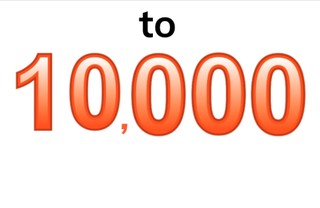Know numbers to 10,000

# Know numbers to 10,000

I can recognize and name numbers up to 10,000.

No account needed.8,000 schools use Gynzy92,000 teachers use Gynzy1,600,000 students use Gynzy

## General

Students learn to recognize numbers from 1,000 to 10,000 and can write the numbers when given a spoken number. They learn and practice the written form, the spoken form and correct notation for these numbers.

## Standards

CCSS.Math.Content..4.NBT.A.2

## Learning objective

Students will be able to recognize and name numbers up to 10,000.

## Introduction

Show the students the numbers on the interactive whiteboard. Ask students to say the given numbers out loud. You can select a student to say a number or ask the class to say the number together.

## Instruction

Point at the thousands numbers to 10,000 and say the numbers aloud. Ask students to repeat the numbers out loud after you say the number. Tell students that you can say thousands numbers in two ways. The number 1,500 can be spoken as one thousand five hundred, or as fifteen hundred. Practice with a few difficult thousands numbers like 6,230 and 8,487. Explain that you do say years differently, even though they are thousands numbers. Distinguish between years up to 2000 and after 2000. Check that students have understood by asking them to match the spoken number to the given numbers. Ask students to write the number on their own sheet of paper or mini whiteboard and then to say the number out loud. Next explain correct notation for thousands numbers. Use the numbers 4725 and 4752 as examples. Remind students that you start with the thousands, place a comma, then the hundreds, tens, and ones. Use the next two example questions to have students write the number. Erase the grey squares on the board to check the student's answers.

Check that students have understood and are able to recognize and name numbers to 10,000 by asking the following questions:
- Where might you run into these numbers?
- Can you give an example of this? (step counter, population of a city, number of visitors, number of objects...)
- Can you explain how you write down a thousands number? For example: 7,650
- How do you say the number 8723? Can you also say it a different way?
- Can you give an example of a year, and spoken like a year?

## Quiz

Students are given a set of questions in which they are either given a number written out that they must write in digits, an audio file that says a number which they must write, or they must take the number in written form from a short text and write the digits.

## Closing

Discuss with students why it is important to be able to know and recognize numbers to 10,000. You see and can find numbers everywhere, and knowing these numbers makes you able to participate with these numbers. For example if you are part of a marathon and they call your number, you can then recognize it. Check that students have understood by asking them to say the numbers on the interactive whiteboard out loud and in their different spoken forms.

## Teaching tips

Students who have difficulty with this learning goal can be supported by spending more time on numbers to 1,000. Students who are ready to be challenged can already work on numbers to 100,000.

### The online teaching platform for interactive whiteboards and displays in schools

• Save time building lessons

• Manage the classroom more efficiently

• Increase student engagement